Courses

# The Hybrid Model For Two Port Network Electrical Engineering (EE) Notes | EduRev

## Electronic Devices

Created by: Cstoppers Instructors

## Electrical Engineering (EE) : The Hybrid Model For Two Port Network Electrical Engineering (EE) Notes | EduRev

The document The Hybrid Model For Two Port Network Electrical Engineering (EE) Notes | EduRev is a part of the Electrical Engineering (EE) Course Electronic Devices.
All you need of Electrical Engineering (EE) at this link: Electrical Engineering (EE)

THE HYBRID MODEL FOR TWO PORT NETWORK:

Based on the definition of hybrid parameters the mathematical model for two port networks known as h-parameter model can be developed. The hybrid equations can be written as: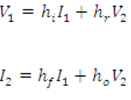(The following convenient alternative subscript notation is recommended by the IEEE Standards), given as:

i=11= input                      = 22 = output

=21 = forward transfer     = 12 = reverse transfer

We may now use the four h parameters to construct a mathematical model of the device of Fig.(1). The hybrid circuit for any device indicated in Fig.(2). We can verify that the model of Fig.(2) satisfies above equations by writing Kirchhoff's voltage and current laws for input and output ports.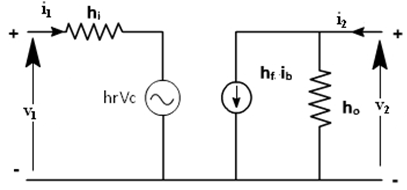If these parameters are specified for a particular configuration, then suffixes e,b or c are also included, e.g. hfe ,hib are h parameters of common emitter and common collector amplifiers.

Using two equations, the generalized model of the amplifier can be drawn as shown in fig. 2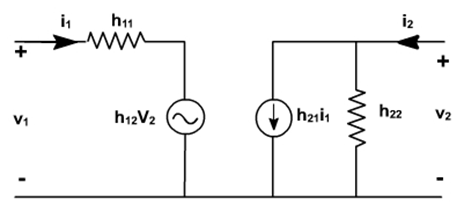fig. 2

TRANSISTOR HYBRID MODEL:

The hybrid model for a transistor amplifier can be derived as follows:

Let us consider CE configuration as shown in fig. 3. The variables, iB, iC ,vC, and vB represent total instantaneous currents and voltages, iB and vC can be taken as independent variables and vB, IC as dependent variables.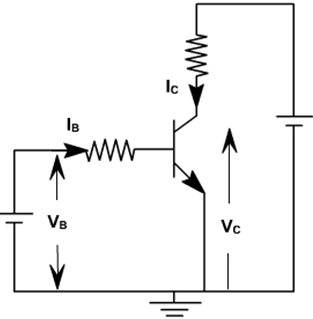fig. 3

VB = f1(iB ,vC )

IC = f2(iB ,vC).

Using Taylor 's series expression, and neglecting higher order terms we get,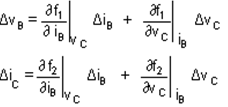The partial derivatives are taken keeping the collector voltage or base current constant. The ΔvB, ΔvC, ΔiB, ΔiC represent the small signal (incremental) base and collector current and voltage and can be represented as vB, iC, iB ,vC.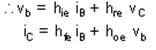where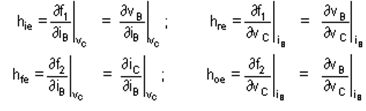The model for CE configuration is shown in fig. 4.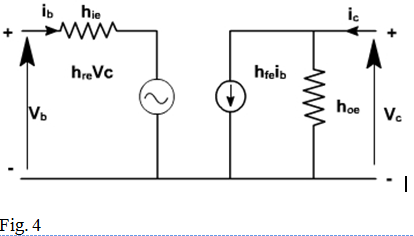To determine the four h-parameters of transistor amplifier, input and output characteristic are used. Input characteristic depicts the relationship between input voltage and input current with output voltage as parameter. The output characteristic depicts the relationship between output voltage and output current with input current as parameter. Fig. 5, shows the output characteristics of CE amplifier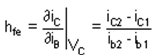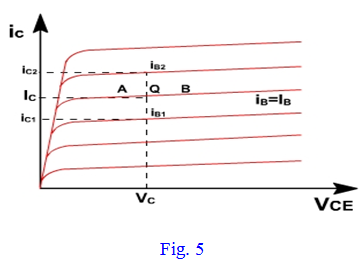The current increments are taken around the quiescent point Q which corresponds to iB = IB and to the collector voltage VCE = VC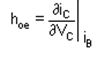The value of hoe at the quiescent operating point is given by the slope of the output characteristic at the operating point (i.e. slope of tangent AB).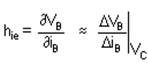hie is the slope of the appropriate input on fig. 6, at the operating point (slope of tangent EF at Q).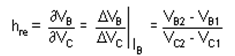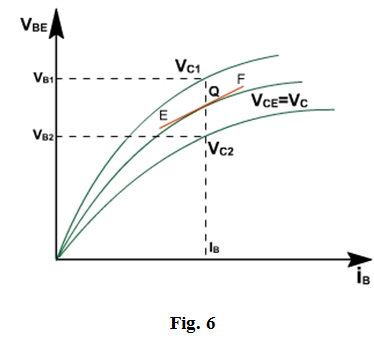A vertical line on the input characteristic represents constant base current. The parameter hre can be obtained from the ratio (VB2– V B1 ) and (VC2– V C1 ) for at Q.

Typical CE h-parameters of transistor 2N1573 are given below:

hie = 1000 ohm.
hre = 2.5 * 10–4
hfe = 50

hoe=25 A/V

Offer running on EduRev: Apply code STAYHOME200 to get INR 200 off on our premium plan EduRev Infinity!

,

,

,

,

,

,

,

,

,

,

,

,

,

,

,

,

,

,

,

,

,

;